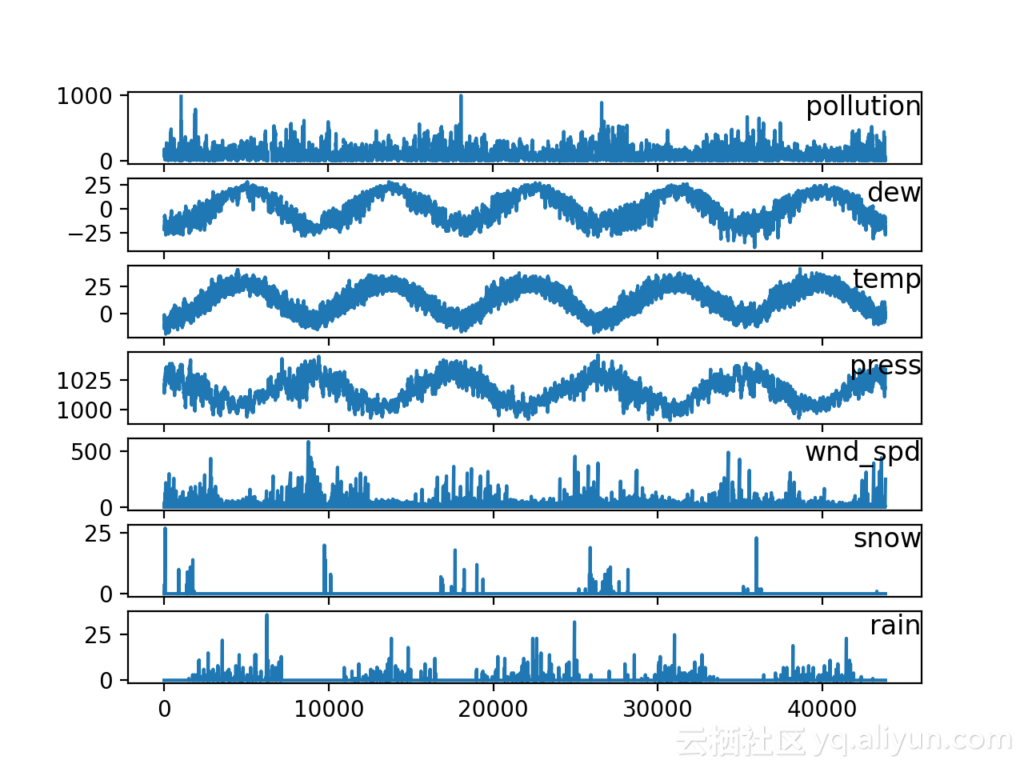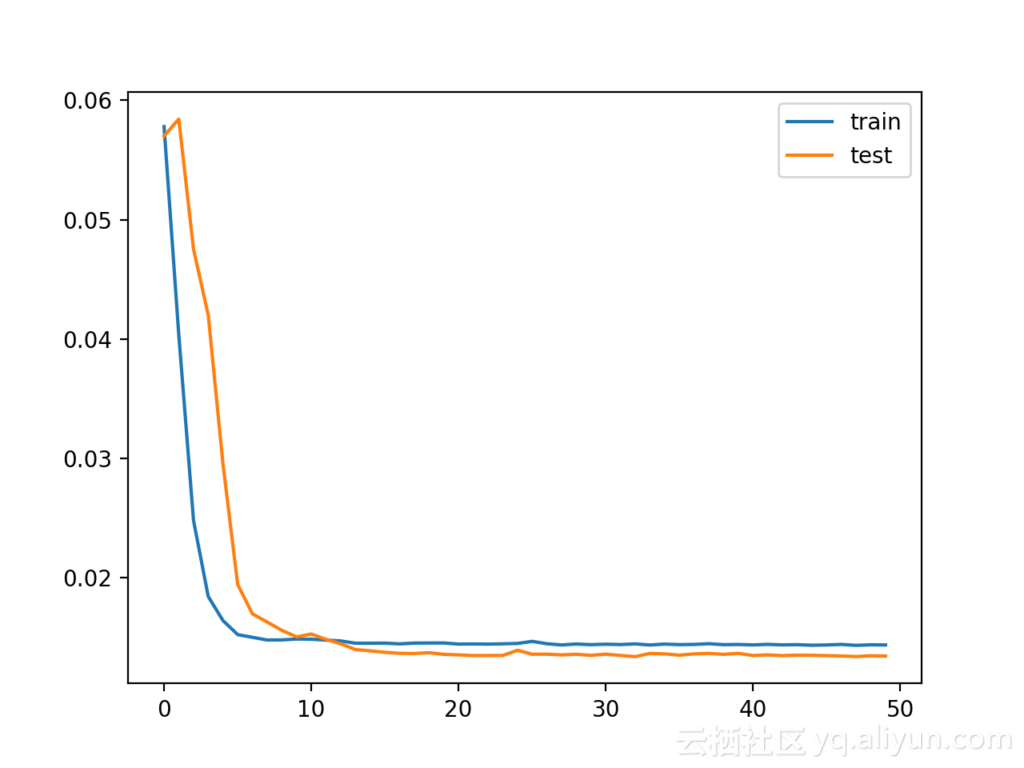#3
6
101. 云栖社区>
2. 博客>
3. 正文

## 基于Keras的LSTM多变量时间序列预测

【方向】 2017-08-17 23:04:31 浏览56465

LSTM是一种时间递归神经网络，它出现的原因是为了解决RNN的一个致命的缺陷。原生的RNN会遇到一个很大的问题，叫做The vanishing gradient problem for RNNs，也就是后面时间的节点会出现老年痴呆症，也就是忘事儿，这使得RNN在很长一段时间内都没有受到关注，网络只要一深就没法训练。后来有些大牛们开始使用递归神经网络来对时间关系进行建模。而根据深度学习三大牛的阐述，LSTM网络已被证明比传统的RNNS更加有效。

·         如何将原始数据集转换为可用于时间序列预测的数据集。

·         如何准备数据并创建适应多变量时间序列预测问题的LSTM

·         如何做出预测并将结果重新调整到原始单位。

1.空气污染预报。

2.基本数据准备。

3.多变量LSTM预测模型。

## 空气污染预报

1.NO：行号。

2.year：年。

3.month：月。

4.day：日。

5.hour：小时。

6.pm2.5PM2.5浓度。

7.DEWP：露点温度。

8.TEMP：温度。

9.PRES：压力。

10.cbwd：风向。

11.Iws：风速。

12.ls：积雪的时间

13.Ir：累积的下雨时数

## 基本数据准备

``````No,year,month,day,hour,pm2.5,DEWP,TEMP,PRES,cbwd,Iws,Is,Ir
1,2010,1,1,0,NA,-21,-11,1021,NW,1.79,0,0
2,2010,1,1,1,NA,-21,-12,1020,NW,4.92,0,0
3,2010,1,1,2,NA,-21,-11,1019,NW,6.71,0,0
4,2010,1,1,3,NA,-21,-14,1019,NW,9.84,0,0
5,2010,1,1,4,NA,-20,-12,1018,NW,12.97,0,0``````

``````from pandas import read_csv
from datetime import datetime
def parse(x):
return datetime.strptime(x, '%Y %m %d %H')
dataset = read_csv('raw.csv',  parse_dates = [['year', 'month', 'day', 'hour']], index_col=0, date_parser=parse)
dataset.drop('No', axis=1, inplace=True)
# manually specify column names
dataset.columns = ['pollution', 'dew', 'temp', 'press', 'wnd_dir', 'wnd_spd', 'snow', 'rain']
dataset.index.name = 'date'
# mark all NA values with 0
dataset['pollution'].fillna(0, inplace=True)
# drop the first 24 hours
dataset = dataset[24:]
# summarize first 5 rows
# save to file
dataset.to_csv('pollution.csv')
``````

`````` Date   				pollution dew temp  press wnd_dir  wnd_spd snow rain
2010-01-02 00:00:00    129.0  -16  -4.0  1020.0    SE    1.79    0     0
2010-01-02 01:00:00    148.0  -15  -4.0  1020.0    SE    2.68    0     0
2010-01-02 02:00:00    159.0  -11  -5.0  1021.0    SE    3.57    0     0
2010-01-02 03:00:00    181.0  -7   -5.0  1022.0    SE    5.36    1     0
2010-01-02 04:00:00    138.0  -7   -5.0  1022.0    SE    6.25    2     0
``````

``````from pandas import read_csv
from matplotlib import pyplot
values = dataset.values
# specify columns to plot
groups = [0, 1, 2, 3, 5, 6, 7]
i = 1
# plot each column
pyplot.figure()
for group in groups:
pyplot.subplot(len(groups), 1, i)
pyplot.plot(values[:, group])
pyplot.title(dataset.columns[group], y=0.5, loc='right')
i += 1
pyplot.show()
``````# 多变量LSTM预测模型建立：

### LSTM数据准备

``````# convert series to supervised learning
def series_to_supervised(data, n_in=1, n_out=1, dropnan=True):
n_vars = 1 if type(data) is list else data.shape
df = DataFrame(data)
cols, names = list(), list()
# input sequence (t-n, ... t-1)
for i in range(n_in, 0, -1):
cols.append(df.shift(i))
names += [('var%d(t-%d)' % (j+1, i)) for j in range(n_vars)]
# forecast sequence (t, t+1, ... t+n)
for i in range(0, n_out):
cols.append(df.shift(-i))
if i == 0:
names += [('var%d(t)' % (j+1)) for j in range(n_vars)]
else:
names += [('var%d(t+%d)' % (j+1, i)) for j in range(n_vars)]
# put it all together
agg = concat(cols, axis=1)
agg.columns = names
# drop rows with NaN values
if dropnan:
agg.dropna(inplace=True)
return agg
values = dataset.values
# integer encode direction
encoder = LabelEncoder()
values[:,4] = encoder.fit_transform(values[:,4])
# ensure all data is float
values = values.astype('float32')
# normalize features
scaler = MinMaxScaler(feature_range=(0, 1))
scaled = scaler.fit_transform(values)
# frame as supervised learning
reframed = series_to_supervised(scaled, 1, 1)
# drop columns we don't want to predict
reframed.drop(reframed.columns[[9,10,11,12,13,14,15]], axis=1, inplace=True)
``````

`````` var1(t-1)    var2(t-1)    var3(t-1)   var4(t-1)    var5(t-1)    var6(t-1)  \
1   0.129779   0.352941   0.245902   0.527273   0.666667   0.002290
2   0.148893   0.367647   0.245902   0.527273   0.666667   0.003811
3   0.159960   0.426471   0.229508   0.545454   0.666667   0.005332
4   0.182093   0.485294   0.229508   0.563637   0.666667   0.008391
5   0.138833   0.485294   0.229508   0.563637   0.666667   0.009912
var7(t-1)  var8(t-1)   var1(t)
1   0.000000    0.0   0.148893
2   0.000000    0.0   0.159960
3   0.000000    0.0   0.182093
4   0.037037    0.0   0.138833
5   0.074074    0.0   0.109658
``````

### 定义和拟合模型

``````# split into train and test sets
values = reframed.values
n_train_hours = 365 * 24
train = values[:n_train_hours, :]
test = values[n_train_hours:, :]
# split into input and outputs
train_X, train_y = train[:, :-1], train[:, -1]
test_X, test_y = test[:, :-1], test[:, -1]
# reshape input to be 3D [samples, timesteps, features]
train_X = train_X.reshape((train_X.shape, 1, train_X.shape))
test_X = test_X.reshape((test_X.shape, 1, test_X.shape))
print(train_X.shape, train_y.shape, test_X.shape, test_y.shape)
``````

``(8760, 1, 8) (8760,) (35039, 1, 8) (35039,)``

``````# design network
model = Sequential()
# fit network
history = model.fit(train_X, train_y, epochs=50, batch_size=72, validation_data=(test_X, test_y), verbose=2, shuffle=False)
# plot history
pyplot.plot(history.history['loss'], label='train')
pyplot.plot(history.history['val_loss'], label='test')
pyplot.legend()
pyplot.show()
``````

### 完整例子``````Epoch 46/50
0s - loss: 0.0143 - val_loss: 0.0133
Epoch 47/50
0s - loss: 0.0143 - val_loss: 0.0133
Epoch 48/50
0s - loss: 0.0144 - val_loss: 0.0133
Epoch 49/50
0s - loss: 0.0143 - val_loss: 0.0133
Epoch 50/50
0s - loss: 0.0144 - val_loss: 0.0133
Test RMSE: 3.836
``````

## 进一步阅读

·北京PM2.5数据集在UCI机器学习库中

·克拉斯长期记忆模型的5步生命周期

·使用Python中的长时间内存网络进行时间序列预测

·Python中的长时间内存网络进行多步时间序列预测

## 总结：

·如何将原始数据集转换为可用于时间序列预测的内容。

·如何准备数据并适应多变量时间序列预测问题的LSTM

· 如何做出预测并将结果重新调整到原始单位。

【方向】
+ 关注